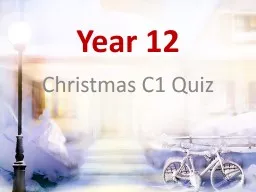# Year 122016-02-27 24K 24 0 0

## Year 12 - Description

Christmas C1 Quiz. Question 1.. What is the gradient of the graph with the equation 2y – 7x – 1 = 0?. Question 2.. What is the gradient of the line from the points (-4, -2) to (-1, -5)?. Question 3.. ID: 233354 Download Presentation

Embed code:

## Year 12

Download Presentation - The PPT/PDF document "Year 12" is the property of its rightful owner. Permission is granted to download and print the materials on this web site for personal, non-commercial use only, and to display it on your personal computer provided you do not modify the materials and that you retain all copyright notices contained in the materials. By downloading content from our website, you accept the terms of this agreement.

### Presentations text content in Year 12

Slide1

Year 12

Christmas C1 Quiz

Slide2

Slide3

Question 1.

What is the gradient of the graph with the equation 2y – 7x – 1 = 0?

Slide4

Question 2.

What is the gradient of the line from the points (-4, -2) to (-1, -5)?

Slide5

Question 3.

What is the equation of the line passing through (-2, 5) and (4, -1)?

Slide6

Question 4.

Rationalise the denominator of:

Slide7

Question 5.

Write as simply as possible:

Slide8

Question 6.

What is the equation of y = 2x² after a translation of ?

Slide9

Question 7.

Write 3x² - 12x + 13 in the form a(

x+b

)² + c

Slide10

Question 8.

Solve:

Slide11

Question 9.

Solve the inequality 14 - x² < 5x

Slide12

Question 10.

Find the equation of the curve y = x³ + x after a translation of

Slide13

Slide14

Question 1.

What is the gradient of the graph with the equation 2y – 7x – 1 = 0?

Slide15

Question 2.

What is the gradient of the line from the points (-4, -2) to (-1, -5)?

-1

Slide16

Question 3.

What is the equation of the line passing through (-2, 5) and (4, -1)?

y = -x + 3

Slide17

Question 4.

Rationalise the denominator of:

Slide18

Question 5.

Write as simply as possible:

Slide19

Question 6.

What is the equation of y = 2x² after a translation of ?

y= 2(x+3)² + 2

Slide20

Question 7.

Write 3x² - 12x + 13 in the form a(x+b)² + c

3(x – 2)² + 1

Slide21

Question 8.

Solve:

x = 9

Slide22

Question 9.

Solve the inequality 14 - x² < 5x

x > 2 and x < 7

Slide23

Question 10.

Find the equation of the curve y = x³ + x after a translation of .Give your answer in the formy = ax³ + bx² + cx +d.

y = x³ - 6x² + 13x - 10

Slide24

Slide25

Question 1.

Slide26

Question 2.

Slide27

Question 3.

Slide28

Question 4.

Slide29

Question 5.

Slide30

Two

Slide31

Question 1.

Slide32

Question 2.

Slide33

Question 3.

Slide34

Question 4.

Slide35

Question 5.

Slide36

Problem Round

5 points each

Slide37

Problem 1.

Three white candles and two black candles can be arranged in a number of ways in a pentagon shaped candelabra.

If the candles are placed at random, find the probability that the three white candles will be adjacent.

Slide38

Problem 2.

Snow White gave each of the elves a new scarf for Christmas. Grumpy, Sneezy and Doc were not happy with the colours and decided to exchange. Grumpy gave his red one to Sneezy in exchange for his yellow scarf. Grumpy then changed with Doc for the green one. Then Sneezy and Doc swapped scarves. Who has which scarf?

Slide39

Problem 3.

1 grandmother1 brother1 grandfather2 sisters2 fathers2 sons2 mothers2 daughters4 children1 father-in-law3 grandchildren1 mother-in-law 1 daughter-in-law

At a family Christmas party, it was possible to count everyone there using the following descriptions:

This gives a total of 23 people.But there were a lot less than that.What is the LEAST number of people that could have been present in the room?

Slide40

Problem Round

Slide41

Problem 1.

Three white candles and two black candles can be arranged in a number of ways in a pentagon shaped candelabra.

If the candles are placed at random, find the probability that the three white candles will be adjacent.

As there are ten possible ways of filling the five candle holders, the probability of three whites being adjacent is 5/10 = 1/2

.

Slide42

Problem 2.

Snow White gave each of the elves a new scarf for Christmas. Grumpy, Sneezy and Doc were not happy with the colours and decided to exchange. Grumpy gave his red one to Sneezy in exchange for his yellow scarf. Grumpy then changed with Doc for the green one. Then Sneezy and Doc swapped scarves. Who has which scarf?

Grumpy has the green one, Doc the red and Sneezy the yellow.

Slide43

Problem 3.

1 grandmother1 brother1 grandfather2 sisters2 fathers2 sons2 mothers2 daughters4 children1 father-in-law3 grandchildren1 mother-in-law 1 daughter-in-law

At a family Christmas party, it was possible to count everyone there using the following descriptions:

This gives a total of 23 people.But there were a lot less than that.What is the LEAST number of people that could have been present in the room?